Last updated: 2021-03-24

Checks: 5 1

Knit directory: thesis/analysis/

This reproducible R Markdown analysis was created with workflowr (version 1.6.2). The Checks tab describes the reproducibility checks that were applied when the results were created. The Past versions tab lists the development history.

Great job! The global environment was empty. Objects defined in the global environment can affect the analysis in your R Markdown file in unknown ways. For reproduciblity it’s best to always run the code in an empty environment.

The command set.seed(20210321) was run prior to running the code in the R Markdown file. Setting a seed ensures that any results that rely on randomness, e.g. subsampling or permutations, are reproducible.

Great job! Recording the operating system, R version, and package versions is critical for reproducibility.

Nice! There were no cached chunks for this analysis, so you can be confident that you successfully produced the results during this run.

Great job! Using relative paths to the files within your workflowr project makes it easier to run your code on other machines.

Tracking code development and connecting the code version to the results is critical for reproducibility. To start using Git, open the Terminal and type git init in your project directory.

This project is not being versioned with Git. To obtain the full reproducibility benefits of using workflowr, please see ?wflow_start.

files = list.files("../data/vector/extraction/", pattern = "ANOM", full.names = T)

data = lapply(files, function(x){
filename = str_split(basename(x), "_")[]
if(length(filename) == 3){
unit = filename
buffer = as.numeric(filename)
var = str_remove(filename, ".gpkg")
} else {
unit = filename
buffer = 0
var =  str_remove(filename, ".gpkg")
}

layers = ogrListLayers(x)
layers = layers[grep("attr_", layers)]
data = do.call(cbind, lapply(layers, function(l){
tmp = st_read(x, layer = l, quiet = TRUE)
names(tmp) = l
tmp
}))

data$id = 1:nrow(data) data %>% as_tibble() %>% mutate(unit = unit, buffer = buffer, var = var) %>% gather("time", "value", -id, -unit, -buffer, -var) %>% mutate(time = str_remove(time, "attr_")) }) data = do.call(rbind, data) str(data) tibble [3,571,200 × 6] (S3: tbl_df/tbl/data.frame)$ id    : int [1:3571200] 1 2 3 4 5 6 7 8 9 10 ...
$unit : chr [1:3571200] "basins" "basins" "basins" "basins" ...$ buffer: num [1:3571200] 100 100 100 100 100 100 100 100 100 100 ...
$var : chr [1:3571200] "AGRANOM" "AGRANOM" "AGRANOM" "AGRANOM" ...$ time  : chr [1:3571200] "2000-01" "2000-01" "2000-01" "2000-01" ...
$value : num [1:3571200] 0.0252 0 0 0 0 ... # 2 Missing Values The resulting dataframe contains roughly 1.8 Mio. rows containing the district-month observations for the two different units of analysis as well as the buffered data. Let’s start looking at the unbuffered data. data %>% group_by(unit, buffer) %>% summarise(N = n(), isna = sum(is.na(value)), isnotna = sum(!is.na(value)), perc = sum(is.na(value)) / n() * 100) # A tibble: 8 x 6 # Groups: unit  unit buffer N isna isnotna perc <chr> <dbl> <int> <int> <int> <dbl> 1 basins 0 486240 720 485520 0.148 2 basins 50 486240 0 486240 0 3 basins 100 486240 0 486240 0 4 basins 200 486240 0 486240 0 5 states 0 406560 720 405840 0.177 6 states 50 406560 0 406560 0 7 states 100 406560 0 406560 0 8 states 200 406560 0 406560 0  # 3 Time Series data %>% filter(buffer==0) %>% mutate(time = as.Date(paste0(time, "-01"))) %>% group_by(time, unit) %>% summarise(value = mean(value, na.rm = T)) %>% ggplot() + geom_line(aes(x=time, y=value, color = unit)) + theme_classic() + labs(y="Precipitation [mm]", x = "Time", color = "Unit of Analysis")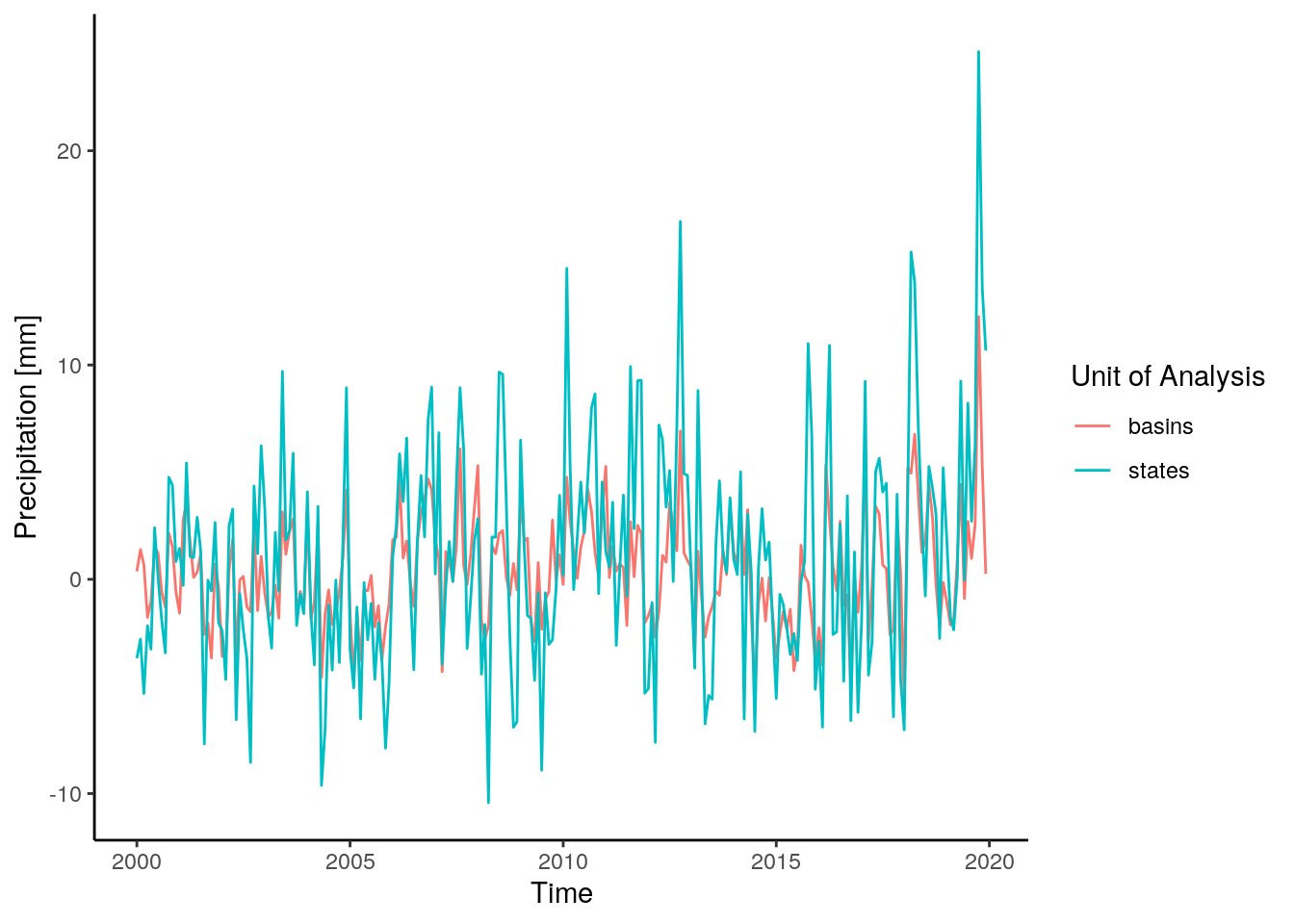# 4 Seasonal decompositon data %>% filter(unit == "basins", buffer == 0) %>% mutate(time = as.Date(paste0(time, "-01"))) %>% group_by(time) %>% summarise(value = mean(value, na.rm = T)) %>% pull(value) %>% ts(start = c(2000,1), frequency = 12) %>% decompose() -> dec_basins data %>% filter(unit == "states", buffer == 0) %>% mutate(time = as.Date(paste0(time, "-01"))) %>% group_by(time) %>% summarise(value = mean(value, na.rm = T)) %>% pull(value) %>% ts(start = c(2000,1), frequency = 12) %>% decompose() -> dec_states dec_data = list(basins = dec_basins, states = dec_states) dec_data = lapply(c("basins", "states"), function(x){ tmp = dec_data[[x]] data.frame(type = x, obsv = as.numeric(tmp$x),
seasonal = as.numeric(tmp$seasonal), trend = as.numeric(tmp$trend),
random = as.numeric(tmp$random), date = seq(as.Date("2000-01-01"), as.Date("2019-12-31"), by = "month")) }) dec_data = do.call(rbind, dec_data) dec_data %>% as_tibble() %>% gather(component, value, -type, -date) %>% mutate(component = factor(component, levels = c("obsv", "trend", "seasonal", "random"))) %>% ggplot() + geom_line(aes(x=date, y=value, color=type)) + facet_wrap(~component, nrow = 4, scales = "free_y") + theme_classic() + labs(y = "Precipitation [mm]", x = "Time", color = "Unit of Analysis") + theme(legend.position="bottom")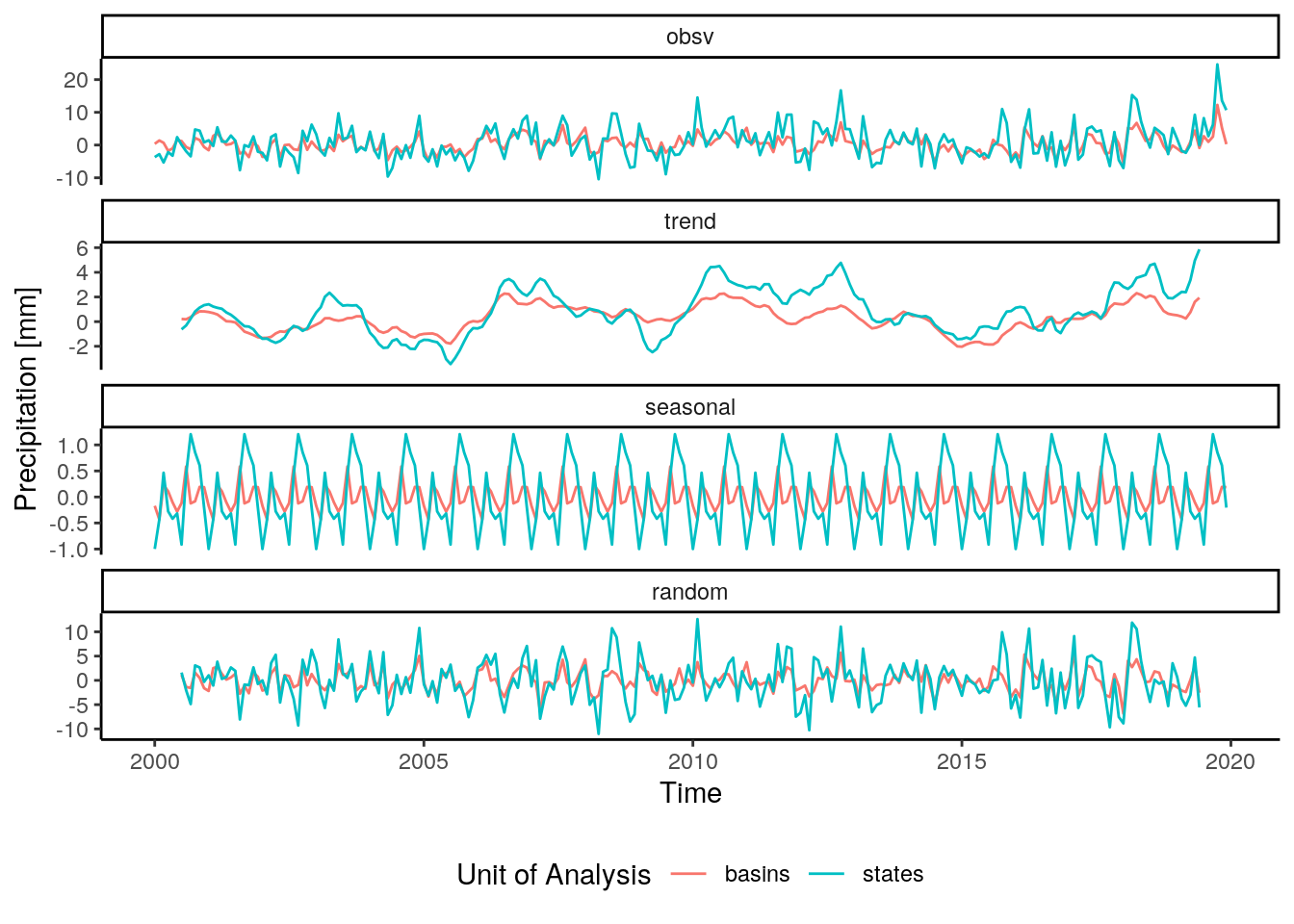# 5 Auto-correlation Analysis data %>% filter( buffer == 0) %>% mutate(time = as.Date(paste0(time, "-01"))) %>% group_by(unit, time) %>% summarise(value = mean(value, na.rm = T)) %>% spread(key = unit, value = value) -> acf_data ## 5.1 States acf(acf_data$states, lag.max = 24)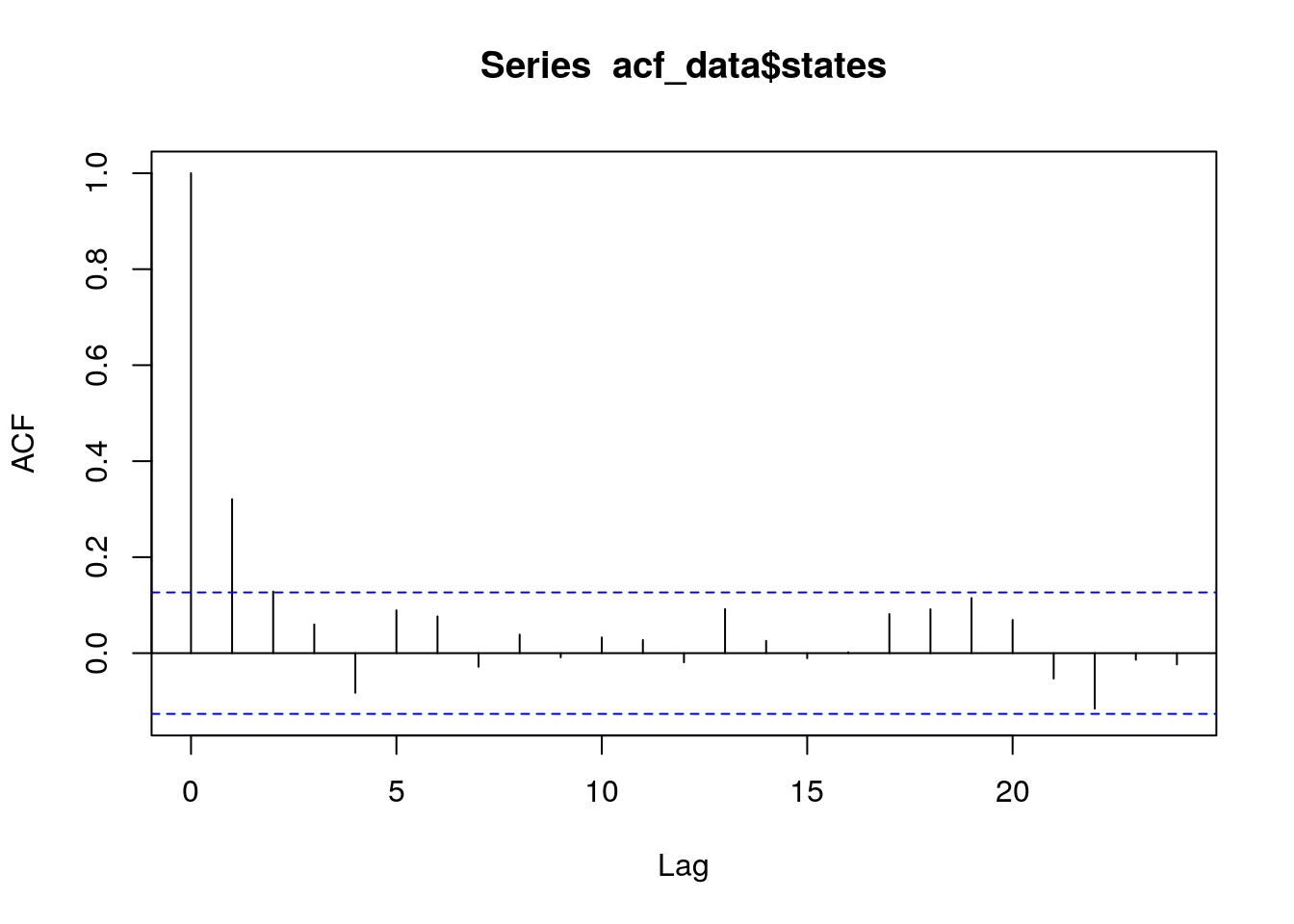acf(acf_data$basins, lag.max = 24)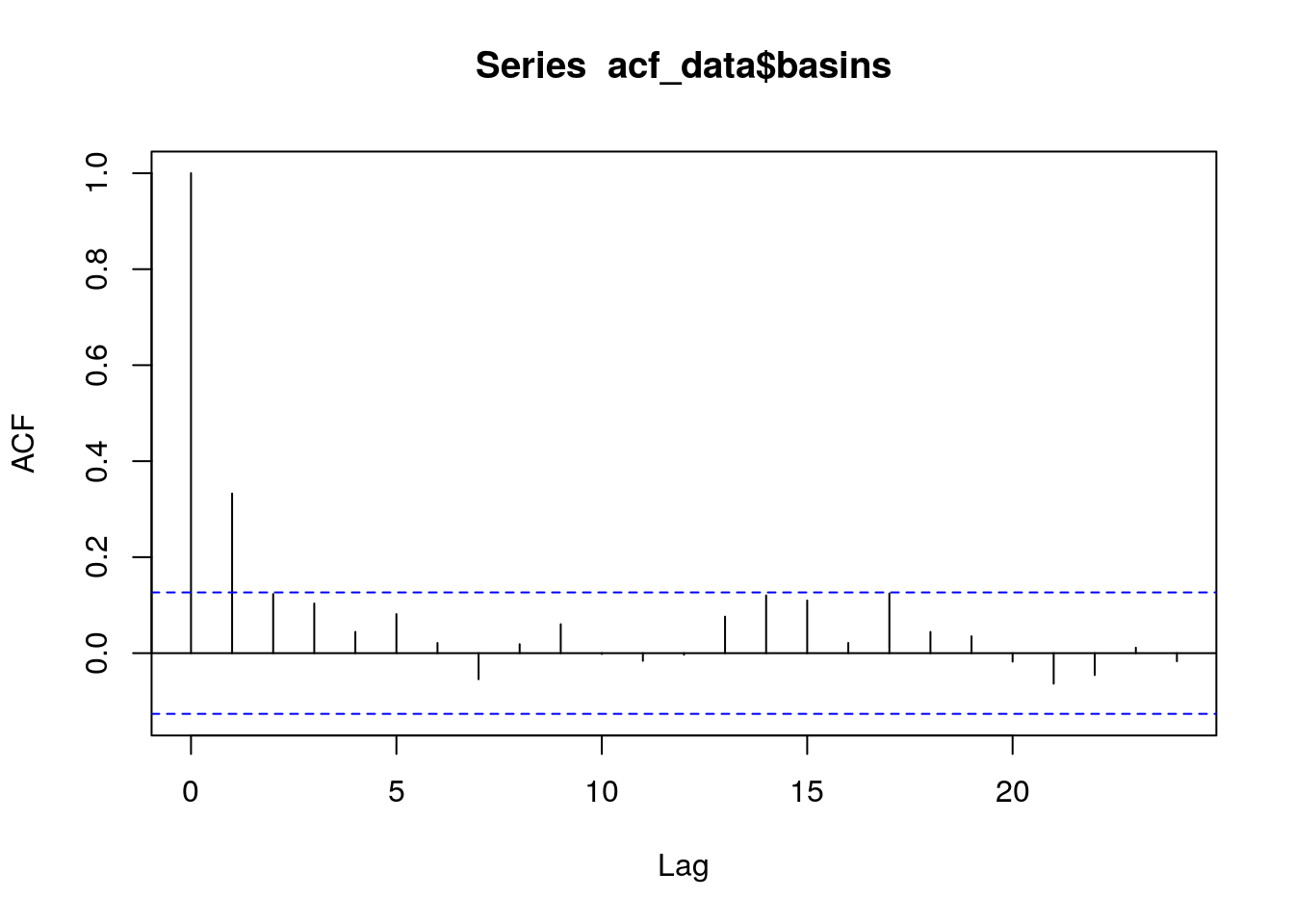# 6 Spatial Pattern poly_bas = st_read("../data/vector/basins_mask.gpkg", quiet = T) crs <- st_crs("EPSG:3857") poly_bas <- st_transform(poly_bas, crs) poly_bas <- st_simplify(poly_bas, dTolerance = 1000, preserveTopology = T) poly_bas$id = 1:nrow(poly_bas)

data %>%
filter(buffer == 0) %>%
mutate(time = as.Date(paste0(time, "-01")),
month = month(time)) %>%
group_by(unit, id, month) %>%
summarise(obsv = sum(is.na(value))) -> obs_data

data %>%
filter(buffer == 0) %>%
mutate(time = as.Date(paste0(time, "-01")),
month = month(time)) %>%
group_by(unit, id, month) %>%
summarise(value = mean(value, na.rm = T)) -> sum_data

poly_bas = left_join(poly_bas, filter(sum_data, unit == "basins"))
poly_bas = left_join(poly_bas, filter(obs_data, unit == "basins"))

## 6.1 Administrative Units by month

### 6.1.1 Number of missing observations

tm_shape(poly_adm) +
tm_polygons("obsv", palette = "-RdBu", border.col = "white", lwd = .5) +
tm_facets("month")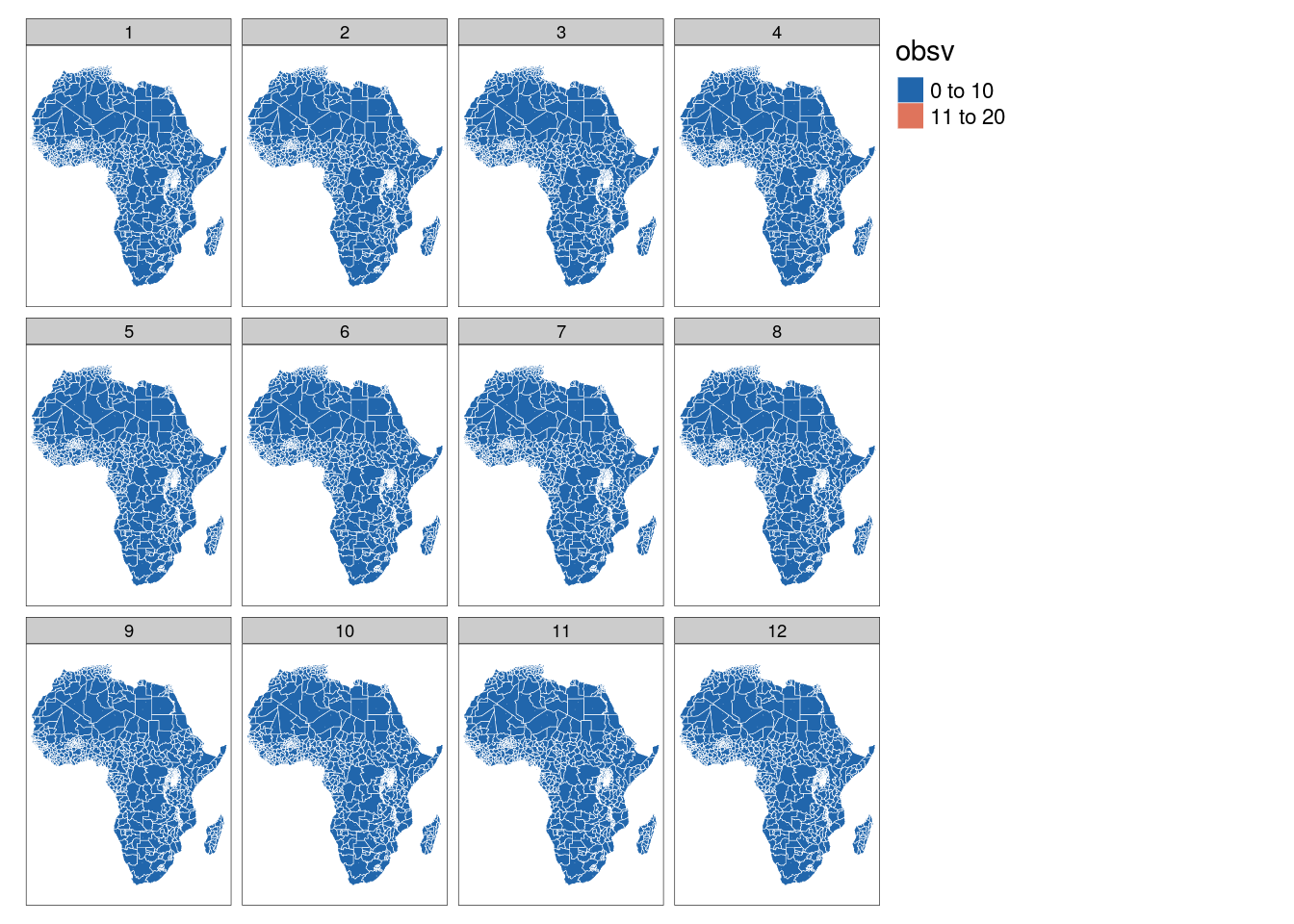### Precipitation map

tm_shape(poly_adm) +
tm_polygons(col = "value", palette = "RdYlBu", breaks = c(-Inf,-30,-20,-10,0,10,20,30,+Inf), border.col = "white", lwd = .5) +
tm_facets("month")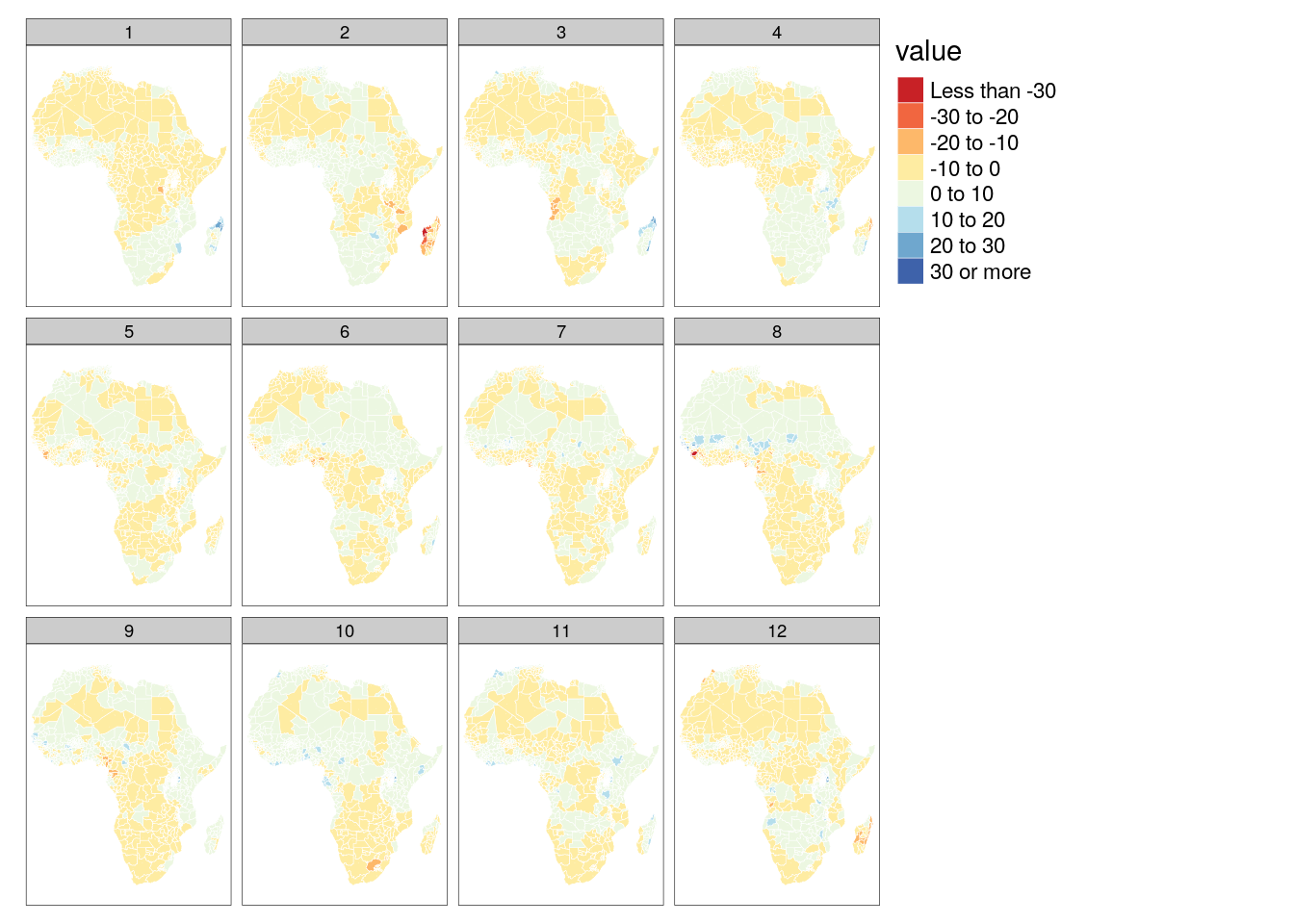## 6.2 Sub-basin watersheds by month

### 6.2.1 Number of missing observations

tm_shape(poly_bas) +
tm_polygons("obsv", palette = "-RdBu", border.col = "white", lwd = .5) +
tm_facets("month")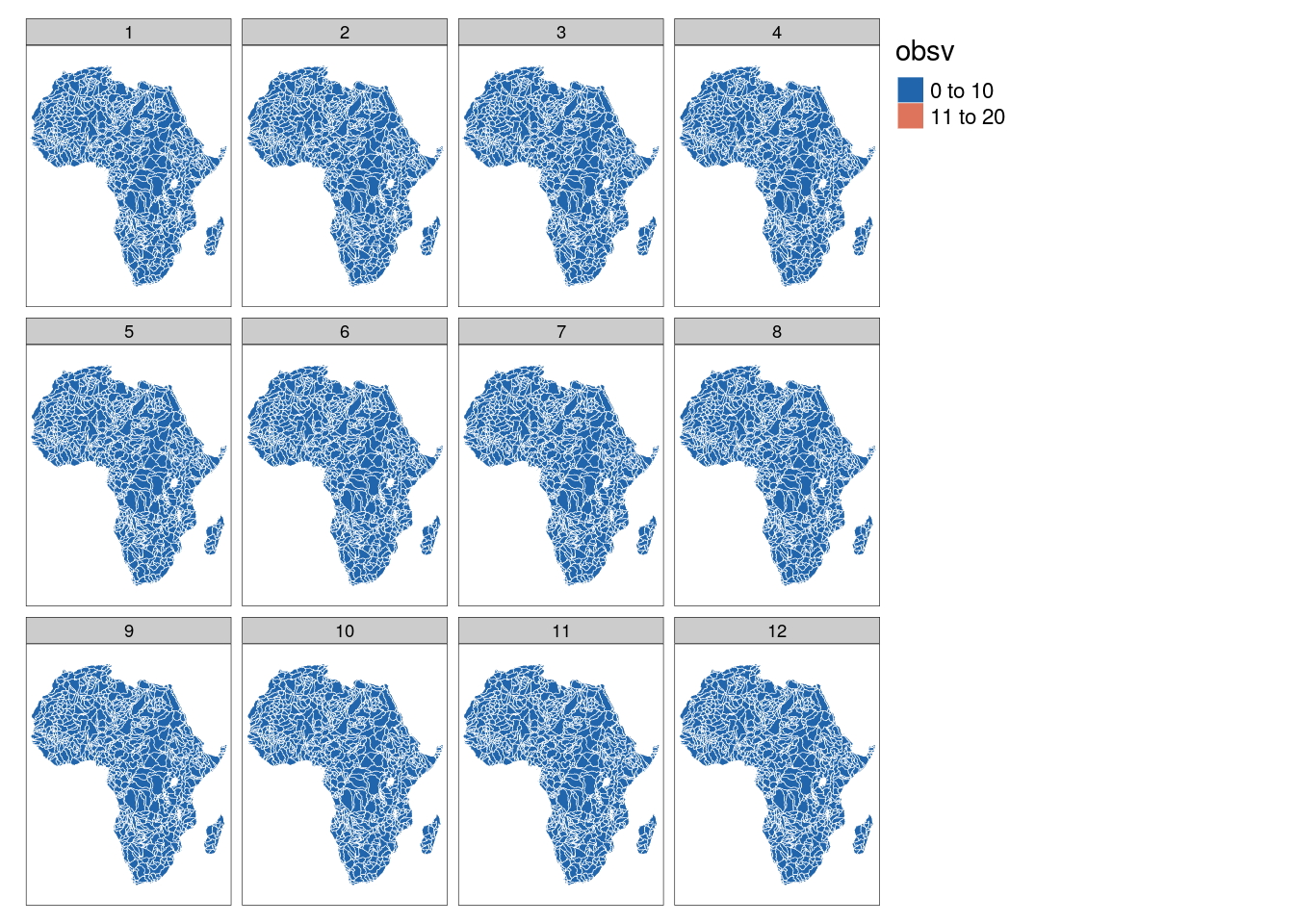### 6.2.2 Precipitation map

tm_shape(poly_bas) +
tm_polygons(col = "value", palette = "RdYlBu", breaks = c(-Inf,-30,-20,-10,0,10,20,30,+Inf), border.col = "white", lwd = .5) +
tm_facets("month")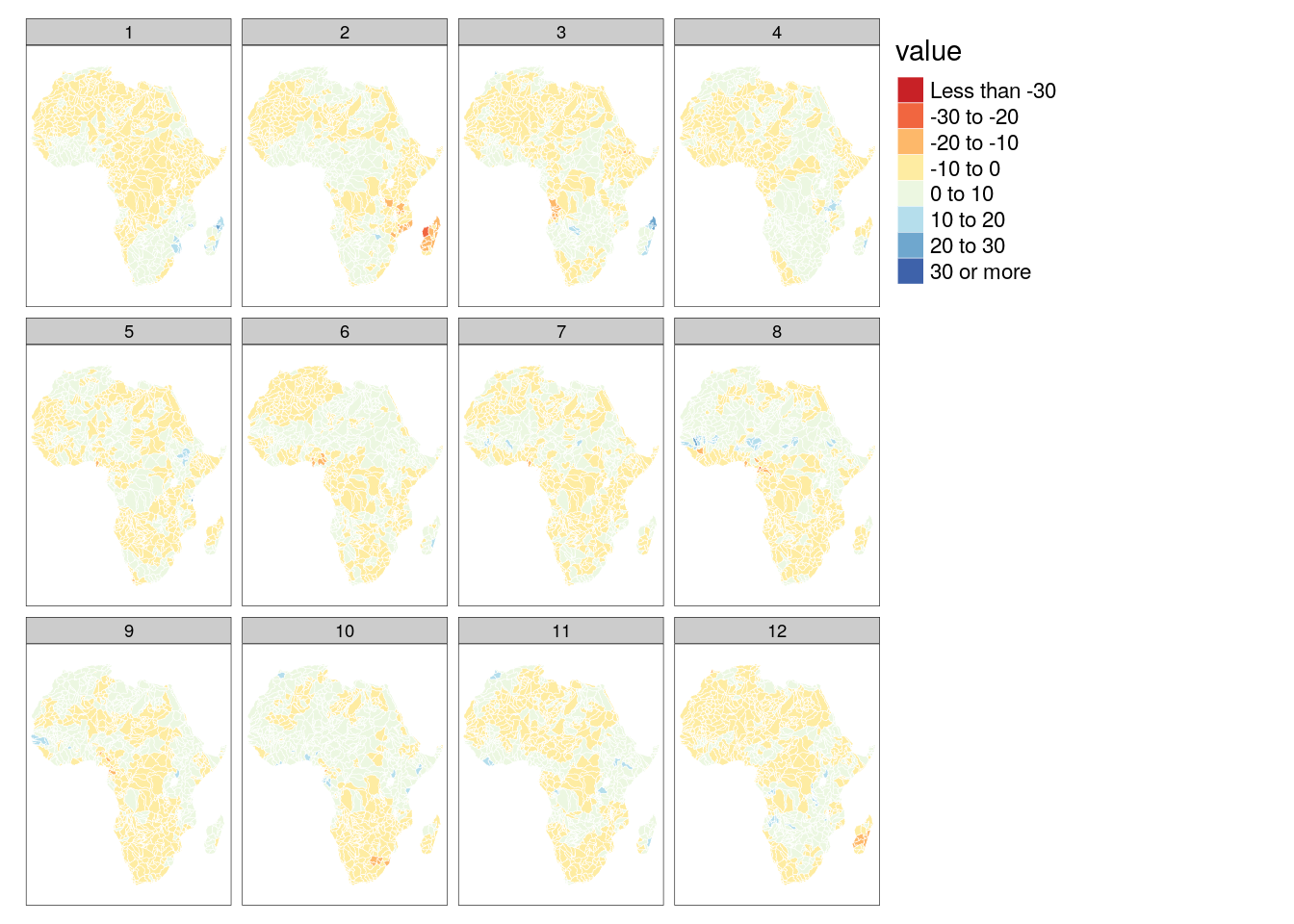sessionInfo()
R version 3.6.3 (2020-02-29)
Platform: x86_64-pc-linux-gnu (64-bit)
Running under: Debian GNU/Linux 10 (buster)

Matrix products: default
BLAS/LAPACK: /usr/lib/x86_64-linux-gnu/libopenblasp-r0.3.5.so

locale:
 LC_CTYPE=en_US.UTF-8       LC_NUMERIC=C
 LC_TIME=en_US.UTF-8        LC_COLLATE=en_US.UTF-8
 LC_MONETARY=en_US.UTF-8    LC_MESSAGES=C
 LC_PAPER=en_US.UTF-8       LC_NAME=C
 LC_MEASUREMENT=en_US.UTF-8 LC_IDENTIFICATION=C

attached base packages:
 stats     graphics  grDevices utils     datasets  methods   base

other attached packages:
 rstatix_0.6.0      ggpubr_0.4.0       scales_1.1.1       RColorBrewer_1.1-2
 latex2exp_0.4.0    cubelyr_1.0.0      gridExtra_2.3      ggtext_0.1.1
 magrittr_2.0.1     tmap_3.2           sf_0.9-7           raster_3.4-5
 sp_1.4-4           forcats_0.5.0      stringr_1.4.0      purrr_0.3.4
 huwiwidown_0.0.1   kableExtra_1.3.1   knitr_1.31         rmarkdown_2.7.3
 bookdown_0.21      ggplot2_3.3.3      dplyr_1.0.2        devtools_2.3.2
 usethis_2.0.0

loaded via a namespace (and not attached):
 lwgeom_0.2-5       splines_3.6.3      crosstalk_1.1.0.1
 leaflet_2.0.3      digest_0.6.27      htmltools_0.5.1.1
 fansi_0.4.2        memoise_1.1.0      openxlsx_4.2.3
 remotes_2.2.0      modelr_0.1.8       prettyunits_1.1.1
 colorspace_2.0-0   rvest_0.3.6        haven_2.3.1
 xfun_0.21          leafem_0.1.3       callr_3.5.1
 crayon_1.4.0       jsonlite_1.7.2     lme4_1.1-26
 glue_1.4.2         stars_0.4-3        gtable_0.3.0
 webshot_0.5.2      car_3.0-10         pkgbuild_1.2.0
 abind_1.4-5        DBI_1.1.0          Rcpp_1.0.5
 viridisLite_0.3.0  gridtext_0.1.4     units_0.6-7
 foreign_0.8-71     htmlwidgets_1.5.3  httr_1.4.2
 ellipsis_0.3.1     farver_2.0.3       pkgconfig_2.0.3
 XML_3.99-0.3       dbplyr_2.0.0       utf8_1.1.4
 labeling_0.4.2     tidyselect_1.1.0   rlang_0.4.10
 later_1.1.0.1      tmaptools_3.1      munsell_0.5.0
 cellranger_1.1.0   tools_3.6.3        cli_2.3.0
 generics_0.1.0     broom_0.7.2        evaluate_0.14
 yaml_2.2.1         processx_3.4.5     leafsync_0.1.0
 fs_1.5.0           zip_2.1.1          nlme_3.1-150
 xml2_1.3.2         compiler_3.6.3     rstudioapi_0.13
 curl_4.3           png_0.1-7          e1071_1.7-4
 testthat_3.0.1     ggsignif_0.6.0     reprex_0.3.0
 statmod_1.4.35     stringi_1.5.3      highr_0.8
 ps_1.5.0           desc_1.2.0         lattice_0.20-41
 Matrix_1.2-18      nloptr_1.2.2.2     classInt_0.4-3
 vctrs_0.3.6        pillar_1.4.7       lifecycle_0.2.0
 data.table_1.13.2  httpuv_1.5.5       R6_2.5.0
 promises_1.1.1     KernSmooth_2.23-18 rio_0.5.16
 sessioninfo_1.1.1  codetools_0.2-16   dichromat_2.0-0
 boot_1.3-25        MASS_7.3-53        assertthat_0.2.1
 git2r_0.27.1       base64enc_0.1-3